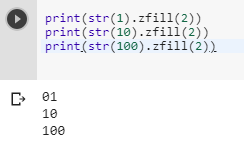3 views
in Python

Given:

a = 1

b = 10

c = 100

How do I display a leading zero for all numbers with less than two digits?

That is,

01

10

100

by (106k points)

In Python, we have a function called str.zfill which can be used to get value with leading zero:

str.zfill():-

This function returns the numeric string left filled with zeros in a string of length width. A sign prefix is handled correctly. The original string is returned if the width is less than or equal to the length of that string.

Following is the code that illustrates how we use it:-

print str(1).zfill(2)

print str(10).zfill(2)

print str(100).zfill(2)